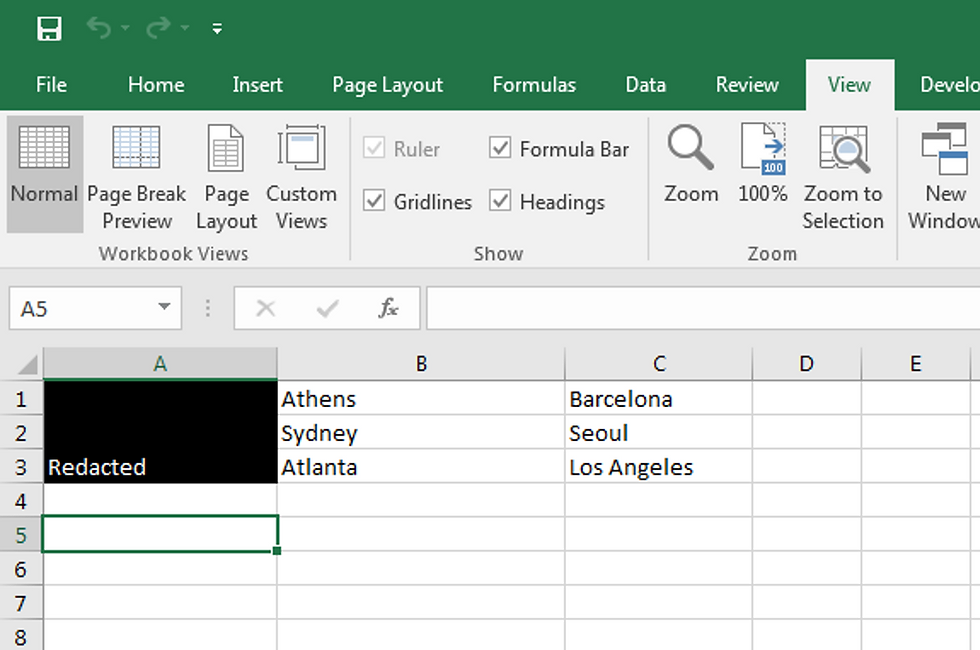Search

# VBA Code to Redact Specified Range in Excel

Here's a little VBA code of my own devising to redact a range of cells in an Excel spreadsheet.

We begin with a spreadsheet that looks like this:Press ALT + F11 and then insert a module with this visual basic code:

Sub textcolor() Range("A1:A3").MergeCells = True Range("A1:A3").Font.Color = vbWhite Range("A1:A3").Interior.ColorIndex = 1 Range("A1").Value = "Redacted" End SubRun the macro and we get a redacted range: